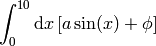# Gauss-Kronrod integration with GSL¶

This is a very simple example to integrate the following expressionusing the Gauss-Kronrod method. This is a non-adaptive method in the sense that function evaluations are not concentrated in the parts of the integration domain which are most significant. The GSL version, will, however employ progressively higher number of function evaluations to obtain the desired estimated accuracy.

## The code¶

Below is the code of a program which computes the above integrand:

// Bojan Nikolic <bojan@bnikolic.co.uk
// Simple Gauss-Konrod integration with GSL

#include <cmath>
#include <iostream>

#include <boost/shared_ptr.hpp>
#include <boost/format.hpp>

#include <gsl/gsl_integration.h>

struct f_params {
// Amplitude
double a;
// Phase
double phi;
};

double f(double x, void *p)
{
f_params &params= *reinterpret_cast<f_params *>(p);
return sin(params.a*x+params.phi);
}

void dointeg(void)
{
f_params params;
params.a=1.0;
params.phi=0.0;

gsl_function F;
F.function = &f;
F.params = reinterpret_cast<void *>(&params);

double result, error;
size_t neval;

const double xlow=0;
const double xhigh=10;
const double epsabs=1e-4;
const double epsrel=1e-4;

int code=gsl_integration_qng (&F,
xlow,
xhigh,
epsabs,
epsrel,
&result,
&error,
&neval);
if(code)
{
std::cerr<<boost::format("There was a problem with integration: code %i") % code
<<std::endl;
}
else
{
std::cout<<boost::format("Result %g +/- %g from %i evaluations") % result % error  % neval
<<std::endl;
}
}

int main(void)
{
dointeg();
}


There are two main parts to this solution:

• Defining the function to be integrated
• Call to GSL routines to carry out the integration

A consequence of using GSL (which is a C-language library) is that it is not possible to define the integrand function with the normal type-safety checks available in C++. This is because GSL requires the function to have a fixed signature:

double f(double x, void *p)


where all of the function parameters besides the variable to be integrated (i.e., the “dummy” variable) are passed through an opaque pointer of type void. It is therefore entirely up to the user to ensure the parameters are passed (through the gsl_function.params variable, below) in the correct format.

In this example we assume that pointer passed to f is in fact a pointer to a structure of type f_params. This is the recommended approach, i.e., passing parameters through a structure rather than as an array of values – it is significantly easier to read/understand and less error prone. Going back to our example, the casting of the void pointer is done in the C++ style by using the reinterpret_cast expression.

The final line of f is the trivial computation of the integrand itself.

The call to GSL to carry out the actual integration is made in the main function. This structure of this call is very straightforward. First the structure of type gsl_function is initialised by specifying the function to integrate and it’s parameters. Then variables are setup that describe the limits of integration and the accuracy desired.

Note also that some non-const variables are created at this stage (result, error and neval). These are used as output variables in the call to GSL by passing their addresses and allowing the GSL routine to write to them. The meaning of result and error are obvious, while neval is the number of evaluations used to get to the required accuracy.